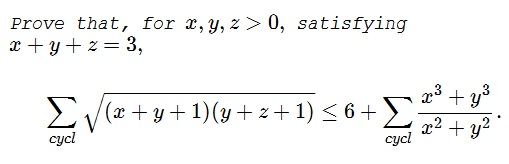Dan Sitaru's Cyclic Inequality in Three Variables IX

ProblemSolution 1

First off, the left-hand side of the inequality is bounded from above via the AM-GM inequality:

\displaystyle \begin{align} LHS&=\sum_{cycl}\sqrt{(x+y+1)(y+z+1)}\le\sum_{cycl}\frac{(x+y+1)+(y+z+1)}{2}\\ &=\sum_{cycl}\frac{x+2y+z+2}{2}=2(x+y+z)+3=9. \end{align}

Thus suffice it to prove that

$\displaystyle 3\le\sum_{cycl}\frac{x^3+y^3}{x^2+y^2}.$

For the right-hand side of the latter inequality we get, via the power-mean inequality:

$\displaystyle \left(\frac{x^3+y^3}{x^2+y^2}\right)^2\ge\frac{x^2+y^2}{2}\ge\left(\frac{x+y}{2}\right)^2,$

so that

$\displaystyle \sum_{cycl}\frac{x^3+y^3}{x^2+y^2}\ge\sum_{cycl}\frac{x+y}{2}=x+y+z=3,$

as required. Equality is attained for $x=y=z=1.$

Solution 2

We refer to an earlier inequality by Nguyen Viet Hung:

Prove that for positive $x,y,z$ subject to $xy+yz+zx=3,$

$\displaystyle \frac{x^3+y^3}{y^2+z^2}+\frac{y^3+z^3}{z^2+x^2}+\frac{z^3+x^3}{x^2+y^2}\le 3.$

Due to the eternal $ab+bc+ca\le a^2+b^2+c^2,$ we get $LHS\le 9.$ It was shown on many occasions (including by Chebyshev) that $9\le RHS.$

Solution 3

From AM-GM,

\displaystyle \begin{align} &\frac{x^3+x^3+y^3}{3}\geq x^2y \\ &\frac{y^3+y^3+x^3}{3}\geq xy^2 . \end{align}

Adding the two inequalities and simplifying,

\displaystyle \begin{align} &x^3+y^3\geq x^2y+xy^2 \\ &2(x^3+y^3)\geq (x^3+y^3)+(x^2y+xy^2)=(x^2+y^2)(x+y) \\ &\frac{x^3+y^3}{x^2+y^2}\geq\frac{x+y}{2} \\ &6+\sum_{cyc}\frac{x^3+y^3}{x^2+y^2}\geq 6+\sum_{cyc}\frac{x+y}{2}=6+3=9~\text{(RHS)}. \end{align}

Applying Cauchy-Schwarz

\displaystyle g\begin{align} \sum_{cyc}\sqrt{(x+y+1)(y+z+1)} &\;\leq \sqrt{\left[\sum_{cyc}(x+y+1)\right]\left[\sum_{cyc}(y+z+1)\right]}\\ &=\sqrt{9\cdot 9}=9~\text{(LHS)}. \end{align}

Thus, $LHS\leq 9 \leq RHS$.

Acknowledgment

Dan Sitaru has kindly posted his problem CutTheKnotMath facebook page. The problem appeared earlier at the Romanian Mathematical Magazine. Solution 2 is by Leo Giugiuc; Solution 3 is by Amit Itagi. Additional solutions can be found at the link.

Inequalities with the Sum of Variables as a Constraint